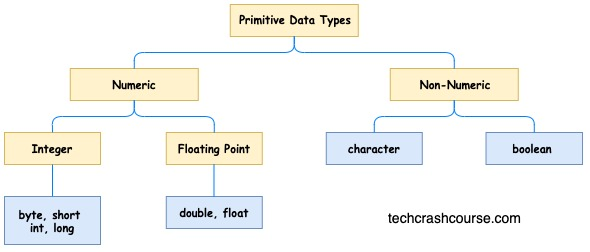# Java Data Types

A data type of a variable in java determines the values it may contain, how much memory is required to store this data, plus the operations that may be performed on it. By creating variables of different data types, we can store integers, floating point numbers, boolean values etc.

Java programming language supports two types of data types primitive and Non-primitive data types. A primitive data type is predefined by the language and is named by a reserved keyword. In this tutorial, we will learn about primitive data types. There are eight primitive data types supported by Java programming language:### byte

• Keyword byte is used to declare variables.
• The byte data type is an 8-bit signed two's complement integer.
• The range of byte data type if from 128 to 127(inclusive).
• We can use variables of byte data types instead of integer data type to save memory. We should use byte data types only when we know the range of values we are dealing with will always be in the range of byte data type.
For Example:
```byte count = 100;
```

### short

• Keyword short is used to declare variables.
• The short data type is a 16-bit signed two's complement integer.
• The range of short data type is from -32,768 to 32,767 (inclusive).
• We can use variables of short data types instead of integer data type to save memory.
• As the range of short data type is more than byte, we can use it to store values beyond the range of byte variables.
For Example:
```short i = -15000, j = 12000;
```

### int

• Keyword int is used to declare integer variables.
• The int data type is a 32-bit signed two's complement integer.
• The range of int data type is from - 2,147,483,648 (-2^31) to 2,147,483,647(2^31 -1)(inclusive).
• We can use variables of int data types if we are not concerned about memory or when you are dealing with number whose values are beyond range of short data types.
For Example:
```int score = 5236521;
```

### long

• Keyword long is used to declare variables.
• The long data type is a 64-bit two's complement integer.
• The range of long data type is from -9,223,372,036,854,775,808(-2^63) to 9,223,372,036,854,775,807(2^63 -1)(inclusive).
• We can use variables of type long when we are dealing with number larger than the range of int data types.
For Example:
```long amount = 645374587L;
```

### float

• Keyword float is used to declare variables.
• The float data type is a single-precision 32-bit IEEE 754 floating point.
• float data type takes less memory than double but float variables cannot store very precise values like double.
For Example:
```float length = 345.6f;
```

### double

• Keyword double is used to declare variables.
• The double data type is a double-precision 64-bit IEEE 754 floating point.
• Double data type is default for decimal numbers.
• double data types takes more memory than float but are more precise than float.
For Example:
```double area = 543.76;
```

### boolean

• Keyword boolean is used to declare variables.
• The boolean data type has only two possible values: true and false.
• We use this variable as a flag to store binary information.
• It stores 1 bit of information but it's actual size is not defined properly.
For Example:
```boolean isComplete = true;
```

### char

• Keyword char is used to declare variables.
• The char data type is a single 16-bit Unicode character.
• The range of char data type is from '\u0000' (0) to '\uffff'(65,535)(inclusive).
• We use char data type to store one character.
For Example:
```char c = 'A';
```

## Default Values of Data Types

It is not compulsory to initialize a variable while declaration. If we don't initialize a variable the compiles assigns a default value as per the data type of the variable. However, it is a good programming practice to initialize a variable during declaration. Here are the default values of various data types:

Data Type Default Value
byte 0
short 0
int 0
long 0L
float 0.0f
double 0.0d
char '\u0000'
boolean false

Here is the Java program to demonstrate the declaration, initialization and printing of primitive data types.

```public class DataTypes {
public static void main(String args[]) {
byte b = 100;
System.out.println("Byte : " + b);

short s = 50;
System.out.println("Short : " + s);

int i = 5236521;
System.out.println("Int : " + i);

long l = 645374587L;
System.out.println("Long : " + l);

float f = 345.6f;
System.out.println("Float : " + f);

double d = 543.76;
System.out.println("Double : " + d);

boolean bl = true;
System.out.println("Boolean : " + bl);

char c = 'A';
System.out.println("Char : " + c);
}
}
```
Output
```Byte : 100
Short : 50
Int : 5236521
Long : 645374587
Float : 345.6
Double : 543.76
Boolean : true
Char : A
```